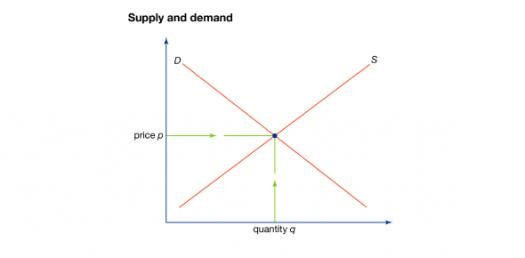# Demand & Supply

10 Questions | Attempts: 16784SettingsThis quiz covers the basics of demand & supply. All questions come from your Introduction to Demand, Introduction to Supply, and Determining Price Notes. You will have 1 minute to answer each question.

• 1.
Which of the following is not a component of demand?
• A.

Desire

• B.

Surplus

• C.

Ability

• D.

Willingness

• 2.
The law of demand states that there is what type of relationship between price and quantity demanded?
• 3.
The demand curve slopes in which direction?
• A.

Down

• B.

Up

• 4.
When each additional unit of a product gives you less utility it is know as what?
• A.

Marginal analysis

• B.

Downward demand

• C.

Diminishing marginal utility

• D.

Decreasing desire

• 5.
The law of supply states there is what type of relationship between price and quantity supplied?
• 6.
The supply curve always slopes in what direction?
• A.

Down

• B.

Up

• 7.
The ideal price level is known as what?
• 8.
A surplus will occur for a product when the price is
• A.

Too high.

• B.

Too low.

• 9.
When there is a shortage what should be done?
• A.

Increase supply

• B.

Decrease price

• C.

Decrease price

• D.

Increase demand

• 10.
When graphing supply and demand, price is always on which axis?
• A.

Horizontal

• B.

Vertical

## Related TopicsBack to top
×

Wait!
Here's an interesting quiz for you.